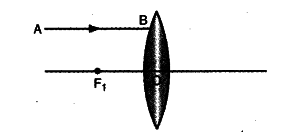# A ray of light AB parallel to the principal axis incident a convex lens as shown in figure

A ray of light AB parallel to the principal axis incident a convex lens as shown in figure. Copy the figure, (i) Draw the refracted ray and (ii) name the point through which the refracted ray passes the principal axis.(i) Refracted ray BD is shown in figure.(ii) The point through which the refracted ray passes the principal axis is known as the principal focus (F_{2} as shown) of the lens.